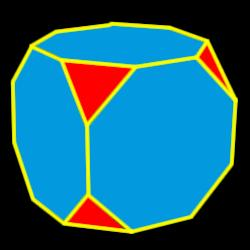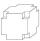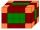# Cube corners

From the cube of edge 5 cm, cut off all vertices so that each cutting plane intersects the edges 1 cm from the nearest vertice.

How many edges will have this body?

n =  36

### Step-by-step explanation:

$n=3\cdot 8+3\cdot 4=36$Did you find an error or inaccuracy? Feel free to write us. Thank you!Tips to related online calculators
Tip: Our volume units converter will help you with the conversion of volume units.

## Related math problems and questions:

• Cube cornersA small cube with an edge length of 2 cm was cut from each corner of a large cube with an edge length of 10 cm. How many cm3 was the body left from the big cube after cutting the small cubes?
• Cube cornersThe wooden cube with edge 64 cm was cut in 3 corners of cube with edge 4 cm. How many cubes of edge 4 cm can be even cut?
• Two boxes-cubesTwo boxes cube with edges a=38 cm and b = 81 cm is to be replaced by one cube-shaped box (same overall volume). How long will be its edge?
• Sphere cutA sphere segment is cut off from a sphere k with radius r = 1. The volume of the sphere inscribed in this segment is equal to 1/6 of the segment's volume. What is the distance of the cutting plane from the center of the sphere?
• Cutting the prismA prism with a square base with a content of 1 cm2 and a height of 3 cm was cut from a cube with an edge length of 3 cm. What is the surface of the body formed from the cube after cutting the prism?
• Magnified cubeIf the lengths of the cube's edges are extended by 5 cm, its volume will increase by 485 cm3. Determine the surface of both the original and the magnified cube.
• Cube cutIn the ABCDA'B'C'D'cube, it is guided by the edge of the CC' a plane witch dividing the cube into two perpendicular four-sided and triangular prisms, whose volumes are 3:2. Determine in which ratio the edge AB is divided by this plane.
• Cutting circlesFrom the square 1 m sides, we have to cut the circles with a radius of 10 cm. How many discs will we cut, and how many percent will be wasted?
• Cube 1-2-3Calculate the volume and surface area of the cube ABCDEFGH if: a) /AB/ = 4 cm b) perimeter of wall ABCD is 22 cm c) the sum of the lengths of all edges of the cube is 30 cm.
• Cubes - diffSecond cubes edge is 2 cm longer than the edge of the first cube. Volume difference blocks is 728 cm3. Calculate the sizes of the edges of the two dice.
• Prism XThe prism with the edges of the lengths x cm, 2x cm, and 3x cm has volume 20250 cm3. What is the area of the surface of the prism?
• Hexagon cut pyramidCalculate the volume of a regular 6-sided cut pyramid if the bottom edge is 30 cm, the top edge is 12 cm, and the side edge length is 41 cm.
• Cuboid wallsCalculate the cuboid volume if its different walls have an area of 195cm², 135cm², and 117cm².
• The cubeThe cube has an edge of 25 cm. We cut it into small cubes of 5 cm long side. How many of these little ones left when we build a new cube of 20 cm in length?
• Volume of three cuboidsCalculate the total volume of all cuboids for which the edges' size are in a ratio of 1:2:3, and one of the edges has a size 6 cm.
• Triangular prism,The regular triangular prism, whose edges are identical, has a surface of 2514 cm ^ 2 (square). Find the volume of this body in cm3 (l).
• HoleIn the center of the cube with edge 14 cm we will drill cylinder shape hole. Volume of the hole must be 27% of the cube. What drill diameter should be chosen?